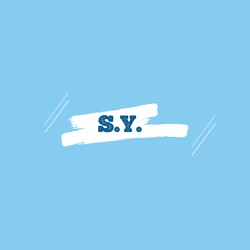# One-legged 2-D Hopping Robot Simulation

Skills: Lagrangian mechanics, impact dynamics, numerical simulations, multi-body systems modeling, Python, variational principles

# Demo

This project models a 2D one-legged hopping robot on a flat ground.# Overview

The motion includes five phases:

• Flight phase 1

The constraint is the length of the leg is at its desired length. Currently, the desired length is set at 0.65 m with a pre-compression of 0.002 m. No external force was added at this stage.

• Touch down impact

The robot experienced a plastic impact. Impact equations include ‘change of momentum lies perpendicular to the contact surface’ and ‘foot arrested to the ground’. Velocity is discontinuous. There is energy loss due to the plastic impact.

• Stance phase

The robot did a passive jump here. The constraint is that the foot is arrested to the ground. The robot took off when the leg length is at its maximum (0.65 m) and hits the mechanical stop.

• Take off impact

Velocity is discontinuous here again. Impact equations include ‘change of momentum lies perpendicular to the contact surface’ and ‘leg is at its maximum length’.

• Flight phase 2

A torque is applied to the hip joint $\theta$ to rotate the leg forward and prepare for the next jump.

A few assumptions were made for simplicity:

• The foot is arrested to the ground during stance (no slip).
• The robot takes off when the leg hits the mechanical stop.
• Impacts are plastic impacts.

The robot has four degrees of freedom:

• Position of the body CoM $x$, $y$
• Orientation of the body $\theta$
• an actuated DoF, a hip rotation torque $\tau$ is applied
• Leg length $l$

This is my final project of ME314 Machine Dynamics (Dec. 2019), NU.

# Multi-body mechanics

This figure (adapted from ) shows the system I'm modeling. This figure shows the frames I'm using.

Rigid body transformations:Specification are listed below. The free length of the spring is $L_{UB} + L_{LB} + \delta$:

• h = 0.25 # leg CG height
• $m_b$ = 3 # body mass
• $m_l$ = 0.3 # leg mass
• $I_b$ = 0.8 # body inertia
• $I_l$ = 0.008 # leg inertia
• $L_{UB}$ = 0.25 # Upper body length
• $L_{LB}$ = 0.15 # Lower body length
• $\delta$ = 0.002 # Spring pre-compression
• $L_L$ = 0.5 # Rigid length of leg
• $K_S$ = 2000 # spring stiffness
• $L_d$ = $L_L$ + $L_{LB}$ # desired leg length

# Future work

• The impact update at the touch-down and take-off phases are manually set as the initial condition of the next phase. An automatic transfer can be implemented.
• The primary purpose of this project is to make the simulation using Lagrangian dynamics. No stable control law is implemented at this stage.

# Reference

•  Raibert, M.H. (1984). Hopping in legged systems — Modeling and simulation for the two-dimensional one-legged case. IEEE Transactions on Systems, Man, and Cybernetics, SMC-14, 451-463.
•  Sayyad, A., Seth, B., & Issac, K.K. (2007). Dynamics and Control of a One-legged 2-D SLOM Hopping Robot.